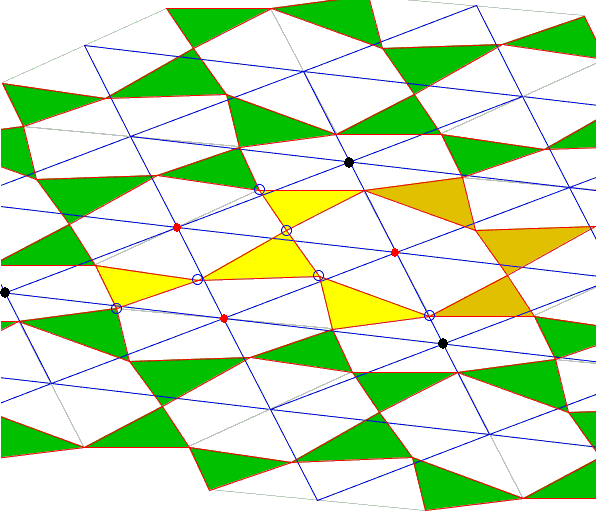# Asymmetric Propeller by Plane Tiling

### Stuart Anderson Oct 2, 2010

Here is a way of seeing the Asymmetric Propeller theorem without using complex numbers. Complex numbers give a much shorter proof, but the following is, I think, a nice visual demonstration.

Let the original triangle have sides a, b, and c, and the three similar copies have the corresponding sides similarly labeled. To produce a tiling, rotate by π the original figure yellow triangles) and attach it to the original as in the applet below (orange triangles). Together, these form one possible unit cell of a tiling. Other obvious unit cells could be formed by attaching the inverted figure in a similar way to other sides of the original figure. (The tiles may overlap for our purposes, since we are not trying to dissect the plane, but to produce a set of translational symmetries. I have chosen triangle orientations that do not produce overlapping cells, purely to make the diagram easier to see.)

### This applet requires Sun's Java VM 2 which your browser may perceive as a popup. Which it is not. If you want to see the applet work, visit Sun's website at https://www.java.com/en/download/index.jsp, download and install Java VM and enjoy the applet.

 What if applet does not run?

Now note that following a connected path consisting of 4 "a" sides moves from a given point to the corresponding point in a neighboring cell, as does a path of 4 "b" sides or 4 "c" sides. Thus the tiling has three periodicities (of which only two are independent of course), and the paths are similar figures proportional in size to the sides a, b, c. Hence corresponding points in three mutually neighboring unit cells form the vertices of a triangle similar to the original one. In particular, consider the three indicated centers (black dots).The tiling consists of triangles and hexagons, and by construction, each hexagon has opposite sides parallel and equal, so the whole lattice has an inversion symmetry around the center of each hexagon. It is therefore obvious that the centers of the three hexagons touching the original triangle (red dots) are the midpoints of the sides of the triangle in the previous paragraph. Hence they themselves for a triangle similar to the original one, and it is also clear that these are the same points the theorem concerns. The theorem follows.

As a point of passing interest, the tiling as a whole shows that each triangle touches exactly three other triangles and three hexagons. Therefore, any of the triangles could be considered the "original one," and there are many more related similarity results. For instance, the centers of any three hexagons which border on a common triangle will form a triangle similar to the original.### Asymmetric Propeller